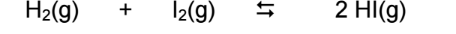# Problem: An equilibrium mixture of H2, I2 and HI at 458°C contains 1.34 atm of H2, 1.34 atm of I2 and 9.30 atm of HI.What is the equilibrium constant (Kp) for this reaction?a) 31.6b) 48.2c) 64.7d) 78.3e) 92.5

🤓 Based on our data, we think this question is relevant for Professor TBA's class at UBC.

###### FREE Expert Solution

We’re being asked to determine the equilibrium constant at 458 ˚C for this reaction:

H2(g) + I2(g)  2 HI(g)

Recall that the equilibrium constant is the ratio of the products and reactants

We use Kp when dealing with pressure and Kc when dealing with concentration:

$\overline{){{\mathbf{K}}}_{{\mathbf{p}}}{\mathbf{=}}\frac{{\mathbf{P}}_{\mathbf{products}}}{{\mathbf{P}}_{\mathbf{reactants}}}}$     $\overline{){{\mathbf{K}}}_{{\mathbf{c}}}{\mathbf{=}}\frac{\mathbf{\left[}\mathbf{products}\mathbf{\right]}}{\mathbf{\left[}\mathbf{reactants}\mathbf{\right]}}}$

Note that solid and liquid compounds are ignored in the equilibrium expression.###### Problem Details

An equilibrium mixture of H2, Iand HI at 458°C contains 1.34 atm of H2, 1.34 atm of I2 and 9.30 atm of HI.

What is the equilibrium constant (Kp) for this reaction?

a) 31.6

b) 48.2

c) 64.7

d) 78.3

e) 92.5What scientific concept do you need to know in order to solve this problem?

Our tutors have indicated that to solve this problem you will need to apply the Equilibrium Expressions concept. If you need more Equilibrium Expressions practice, you can also practice Equilibrium Expressions practice problems.

What is the difficulty of this problem?

Our tutors rated the difficulty ofAn equilibrium mixture of H2, I2 and HI at 458°C contains 1....as medium difficulty.

How long does this problem take to solve?

Our expert Chemistry tutor, Sabrina took 2 minutes and 36 seconds to solve this problem. You can follow their steps in the video explanation above.

What professor is this problem relevant for?

Based on our data, we think this problem is relevant for Professor TBA's class at UBC.